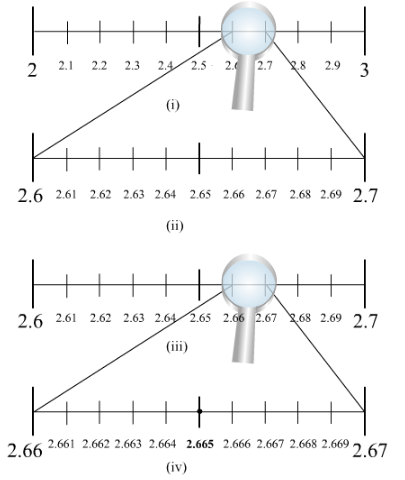# Visualise 2.665 on the number line,Question:

Visualise 2.665 on the number line, using successive magnification.

Solution:We know that 2.665 lies between 2 and 3. So, we divide the number line into 10 equal parts
and mark each point of division. The first mark on the right of 2 will be 2.1 followed by 2.2 and so on.
The point left of 3 will be 2.9. Now, the magnified view of this will show that 2.665 lies between 2.6 and
2.7. So, our focus will be now 2.6 and 2.7. We divide this again into 10 equal parts. The first part will be
2.61 followed by 2.62 and so on.
We now magnify this again and find that 2.665 lies between 2.66 and 2.67. So, we magnify this portion
and divide it again into 10 equal parts. The first part will represent 2.661, next will be 2.662 and so on.
So, 2.665 will be 5th mark in this subdivision as shown in the figure.